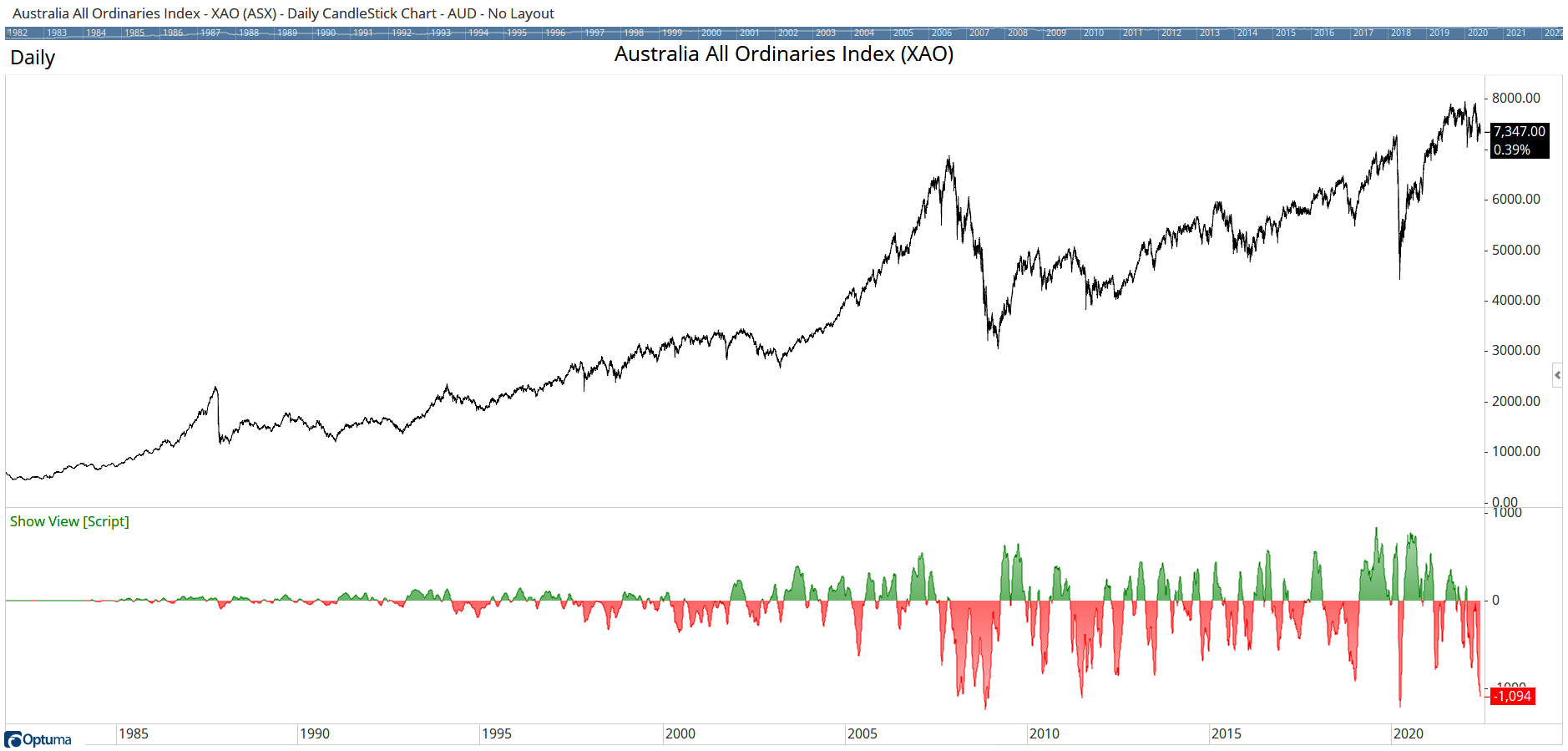Select Page

# ASX McClennan Summation Index

Optuma Forums Optuma Scripting ASX McClennan Summation Index

Viewing 2 posts - 1 through 2 (of 2 total)
• Author
Posts
• #68505

I am using the following script for the ASX McClennan Summation Index.  It works OK for the NYSE but on the ASX it gives no reading before May 2015.  I can’t see why it does this as the ASX was operating well before that date and the code is identical to the NYSE version, except for the data input.

//Source Data for ASX Advance and Declines
D1 = GETDATA(CODE=ADVASX:ASX, CURRENCY=AUD);
D2 = GETDATA(CODE=DECASX:ASX, CURRENCY=AUD);
//Setup 19EMAs on AD Codes
A1 = MA(D1, BARS=19, STYLE=Exponential);
A2 = MA(D2, BARS=19, STYLE=Exponential);
//Setup 39EMAs on AD Codes A3 = MA(D1, BARS=39, STYLE=Exponential); A4 = MA(D2, BARS=39, STYLE=Exponential);
MO=(A1 – A2) – (A3 – A4);
ACC(MO)

I hope you can assist me… thanks  KM

#68509

Hi Keith,

Use the advance / decline data from Breadth Measures dataset rather than ASX. Change D1 to GETDATA(CODE=ADVASX:BM); (and D2 to DECASX:BM) and it will then go back further.

Also, no need to convert to AUD as there is no currency with this data – it’s a simple count.Finally, please remember to format the script text in posts so they can be easily copied in to the software. See the pinned post above: